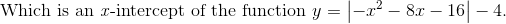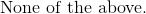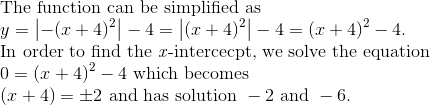# Algebra 1 : How to graph an absolute value function

## Example Questions

### Example Question #1 : How To Graph An Absolute Value Function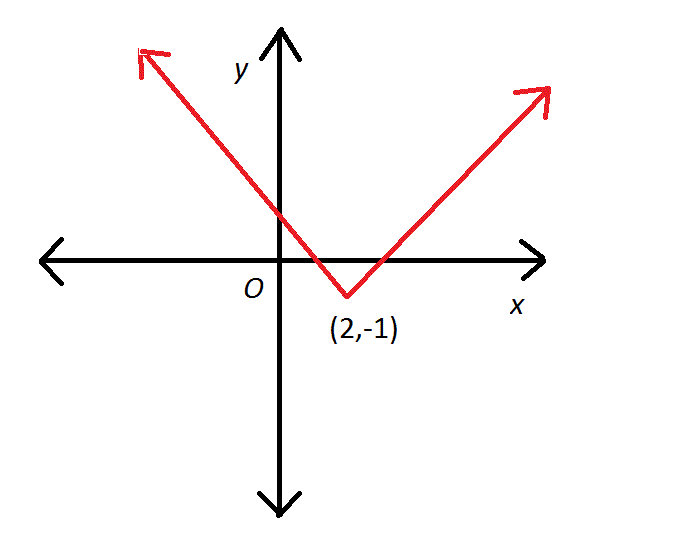Which of these would most likely be the equation corresponding to the above graph?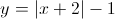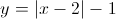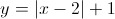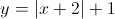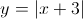Explanation:

This is an absolute value graph. Its equation takes the form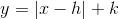, in which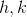represent the number of units that the base graph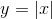is translated right and up respectively.

Since the graph ofis translated two units right and one unit down,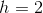and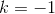, so the equation would be: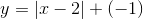or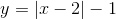### Example Question #2 : How To Graph An Absolute Value Function

Give the-intercept(s) of the graph of the function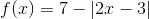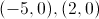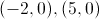The graph has no-intercepts.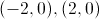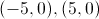Explanation:

To find the-intercept(s) of the graph, set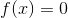and solve for.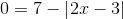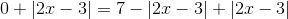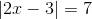Rewrite this as the compound equation: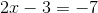or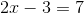Solve each separately: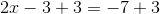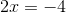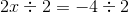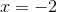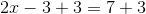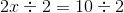There are two-intercepts: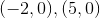### Example Question #3 : How To Graph An Absolute Value Function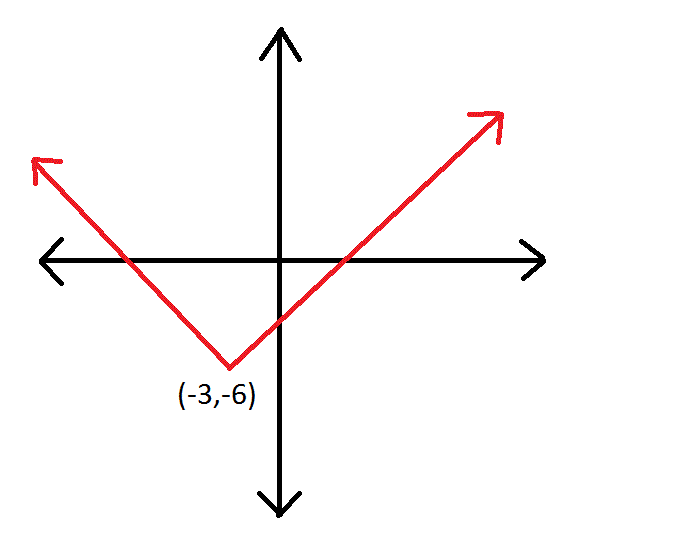Which of these would most likely be the equation corresponding to the above graph?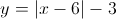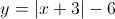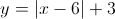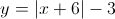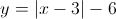Explanation:

This is an absolute value graph. Its equation takes the form, in whichrepresent the number of units that the base graphis translated right and up respectively.

Since the graph ofis translated three units left and six units down,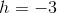and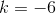.

Plug these values into the general form of the equation: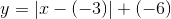Simplify: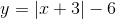### Example Question #41 : Graphing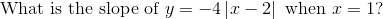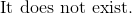Explanation: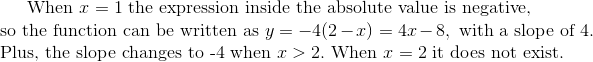### Example Question #5 : How To Graph An Absolute Value Function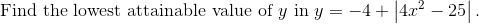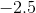Explanation: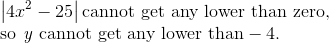### Example Question #1 : How To Graph An Absolute Value Function